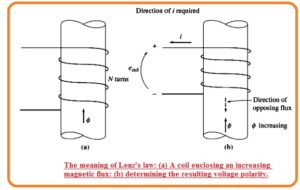Hello friends I hope you all are doing great. In this post, we will discuss Faraday’s Law-Induced Voltage From Time-Changing Magnetic Field. According to Faraday’s law, the voltage induced in the conductive object is equal to the rate of change of flux from that circuitry. If the change in a magnetic field is with a speed that the voltage induced will be large.

In this tutorial, we learn how faraday law effect with the time-changing magnetic field. So let’s get started. Faraday’s Law-Induced Voltage From Time-Changing Magnetic Field

## Faraday’s Law-Induced Voltage From Time-Changing Magnetic Field

• Here the main thing we discuss is the numerous techniques that explain the effect of the field at its near-space The main factor which is observed is Faraday Law.
• The basic operation of the transformer lies at the Faraday law. That explains that if there is flux change in the windings the voltage produced in those windings has a direct relation with the rate of change of flux change according to time.• In this equation, eind denotes the voltage produced in the windings and ø denotes the flux in that windings.
• If there is N number of turns in that windings and flux moving through all turns is similar then voltage produced about the windings is given here.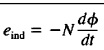Here

• eind denotes voltage production  in the windings
• N is the number of turns coil
• ø flux in that windings
• The minus sign in that equation is due to Lenz law. That defines that direction of voltage generation in the windings is that if windings end pints are linked to each other then-current generated will results in restrction or opposing the flux variation.
• As the induced voltage opposes the variation which results to add minus sign in the above equation.
• For the understanding of this concept see the below-shown figure.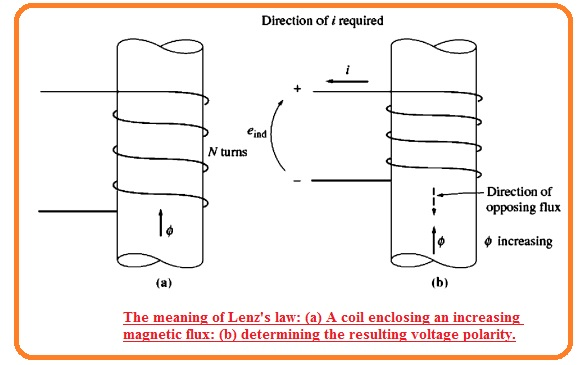• If the total flux in this figure is rising in case of strength then the voltage generation in the windings causes to create a flux that restricts or opposes the increment.
• The current passing can be seen in the figure denoted as b will generate the flux in opposing the increment.
• Therefore voltage of windings should create such polarity which needed to run that current through exterior circuitry.
• So the voltage should be created according to polarity shown as the polarity of the resultant voltage can find through different parameters and minus signs shown in the above equation normally ignored.
• There is one main disturbance that exists in the use of the above equation. This equation says that there is similar quantity of flux exist in the windings turn.
• There is reasons that provide this statement wrong that is leakage flux. If coil windings are fixed very tight then flux through one turn will also pass from the other turn and satisfied above equation.
• If there is a high value of flux leakage with that high-value correctness is needed. The value of voltage in the turn is given here.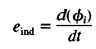• If there is N turns in the windings then the net voltage will be.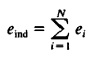• The terms shown in the above equation parentheses is known as flux linkage λ in the windings and Faraday law will be now.• Faraday law is the basic feature of the magnetic field that exists in the transformer. The effect of Lenz law in a transformer helps to know the polarity of voltage generated in the windings of the transformer.
• This law also defines the eddy current losses. The time-varying flux produced voltage in the ferromagnetic cores in a similar way as wire wounded about the core.
• This voltage results in a huge amount of current passing in the core. Due to eddy, current power loss exist that caused heating
• The quantity of power loss through eddy current is in direct relation to the area of that is followed by that current.
• Due to this cause, it is necessary to use lamination in the core after making it in the form of stips.
• There can be use of resin among that strips. Since the insulation sheet is thinner.
• The quantity of eddy current is in direct relation to the square of the thickness of the lamination

That is a detailed post about Faraday’s Law-Induced Voltage From Time-Changing Magnetic Field if you have any further query ask in comments. Thanks for reading have a nice day.